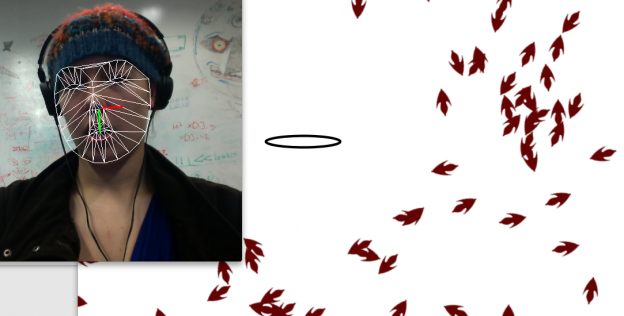# Little FishSummary: A Processing and FaceOSC program featuring tiny fish Abstract: Using the user's webcam, this program tracks the location of the user's mouth in the context of a school of fish. The fish flee upon encountering the user's mouth.

FaceOSC

I’ve been interested in simple life simulation for a while so I used this project as an opportunity to experiment with it. The program begins in Processing by spawning a school of fish. Each fish has the ability to navigate its environment, to turn around at the edges of visible space, and to flee when it gets too close to the user’s mouth. The program then tracks the position of the user’s mouth using FaceOSC, mirroring its size and position in the fish tank. When a fish gets too close to the mouth, it will turn and swim at great speed as far from the mouth as it can get.

There are a lot of areas that I’d like to put some more work into–I was working on implementing a flocking function but couldn’t get it to flow the way I wanted to (the commented code is available on Github). I’d also like to add an element of curiosity to the fish: they’ll run from the mouth, yes, but once they forget their shock, what happens if they follow it? I’ve got a good base to work with but I’ve got a long way to go in making them more convincingly real.

Below is the current code that drives each fish:

```class Fish {
PImage img;
int imgsize = 50;
int xpos;
int ypos;
int xsize = 1000;
int ysize = 800;
float xVelo;
float yVelo;

int avoidDist = 10;
float nosePos;
boolean flipFish;
float turnDeg;

Fish() {
xpos = int(random(10, xsize-20));
ypos = int(random(10, ysize-20));
nosePos = random(0, TWO_PI);
xVelo = 1.0;
yVelo = 1.0;
}
void drawFish() {
pushMatrix();
checkBoundaries();
translate(xpos, ypos);
rotate(nosePos);
image(img, 0, 0);
popMatrix();

if (flipFish == true) {
nosePos += 0.09;
turnDeg += 0.09;
xVelo += 1;
yVelo += 1;
if (turnDeg > PI) {
turnDeg = 0;
xVelo = 1;
yVelo = 1;
flipFish = false;
}
}
}

void trackFaceMovement(Face userFace) {
float xDistFromFace = abs(userFace.posePosition.x - xpos - userFace.mouthWidth);
float yDistFromFace = abs(userFace.posePosition.y - ypos + 20 - userFace.mouthWidth);

fill(#CCCCCC);
if (xDistFromFace < 160 & yDistFromFace < 160) {       flipFish = true;     }   } void moveSemiRandomly(Fish[] f) {   float noseAngle = nosePos;   float yDist = sin(noseAngle);   float xDist = cos(noseAngle);   int xAdd = int(map(xDist, -1, 1, -5, 5));   int yAdd = int(map(yDist, -1, 1, -5, 5));   ypos += (yAdd * yVelo);   xpos += (xAdd * xVelo); } void checkBoundaries() {   boolean flag = false;   if (xpos >= (xsize + 20)) {
xpos = xsize;
flag = true;
xVelo = 1.0;
yVelo = 1.0;
}
else if (xpos < = -40) {     xpos = -40;     flag = true;     xVelo = 1.0;     yVelo = 1.0;   }   else if (ypos >= (ysize + 20)) {
ypos = ysize;
flag = true;
xVelo = 1.0;
yVelo = 1.0;
}
else if (ypos < = 0) {
ypos = 0;
flag = true;
xVelo = 1.0;
yVelo = 1.0;
}
if(flag == true) {
nosePos -= PI;
}
}
};```
This entry was posted in face-osc, project-1 on by .